## Intermediate Python for Data Science: Logic, Control Flow and FilteringBoolean logic is the foundation of decision-making in Python programs. Learn about different comparison operators, how to combine them with Boolean operators, and how to use the Boolean outcomes in control structures. Also learn to filter data in pandas DataFrames using logic.

In the earlier days when I started to learn Python, there is a topic on Boolean and Comparison Operators, where I studied Boolean (True and False), logical operators (‘and’, ‘or’, ‘not’) and comparison operators (‘==’ ‘!=’, ‘<‘ and ‘>’).

Comparison operators can tell how two Python values relate and result in a Boolean. It allows to compare two numbers, strings or any same type of variables. It throws exception or error message when it is comparing a variable from a different data type. Python cannot tell how the two objects of different type relate.

## Comparison a Numpy array with an integer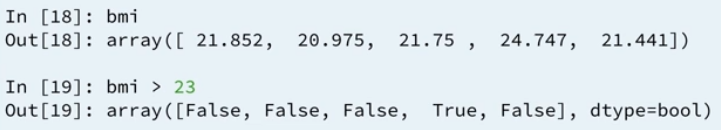Based on the example above taken from a tutorial in DataCamp online learning course that I am taking currently, the variable bmi is a Numpy array, then it compares if the bmi is greater than 23. It works perfectly and returns the Boolean values. Behind the scenes, Numpy builds a Numpy array of the same size, perform an element-wise comparison, filtered with the number 23.

## Boolean operators with Numpy

To use these operators with Numpy, you will need np.logical_and(), np.logical_or() and np.logical_not(). Here’s an example on the my_house and your_house arrays from before to give you an idea:

```np.logical_and(your_house &gt; 13,
your_house < 15)
```

Refer to below for the sample code:

```# Create arrays
import numpy as np
my_house = np.array([18.0, 20.0, 10.75, 9.50])
your_house = np.array([14.0, 24.0, 14.25, 9.0])

# my_house greater than 18.5 or smaller than 10
print(np.logical_or(my_house &gt; 18.5, my_house < 10))

# Both my_house and your_house smaller than 11
print(np.logical_and(my_house < 11, your_house < 11))
```

The first print statement is checking on the ‘or’ condition means, any one of the two condition is true, it returns true. The second print statement is checking on the ‘and’ condition means, both of the comparison has to be True then it returns a True. The output of the execution returns in Boolean array as below:

```[False  True False  True]
[False False False  True]
```

## Combining Boolean operators and Comparison operators with conditional statement, if, else and elif.

It follows the if statement syntax. The most simplest code which can be used to explain the above,

```z = 4
if z % 2 == 0:
print('z is even')
```

Same goes to the if else statement with comparison operator, see code below:

```z = 5
if z % 2 == 0:
print('z is even')
else:
print('z is odd')
```

Or if you are working with if, elif and else statement, it works too. See the code below:

```z = 6
if z % 2 == 0:
print('z is divisible by 2')
elif z % 3 == 0:
print('z is divisible by 3')
else:
print('z is neither divisible by 2 nor 3')
```

In the example above, both first and second condition are matched, however, in this control structure, once Python hits into a condition that returns a True value, it executes the corresponding code and exits the control structure after that. It will not execute the next condition, corresponding to the elif statement.

## Filtering Pandas DataFrame

For an example taken from DataCamp’s tutorial, using the DataFrame below, select countries with area over 8 millions km. There are 3 steps to achieve this.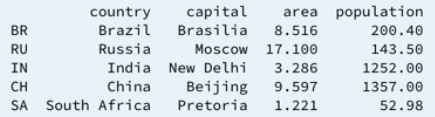Step 1: select the area column from the DataFrame. Ideally, it gets a Pandas Series, not a Pandas DataFrame. Assume that the DataFrame is called bric, then it calls the column area using,

```brics["area"]

#alternatively it can use the below too:
# brics.loc[:, "area"]
# brics.iloc[:, 2]
```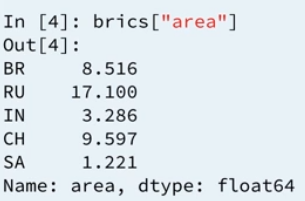Step 2: When the code adds in the comparison operator to see which rows have an area greater than 8, it returns a Series containing Boolean values. The final step is using this Boolean Series to subset the Pandas DataFrame.

Step 3: Store this Boolean Series as ‘is_huge’ as below:

```is_huge = brics["area"] > 8
```

Then, creates a subset of DataFrame using the following code and the result returns as per the screenshot:

```brics[is_huge]
```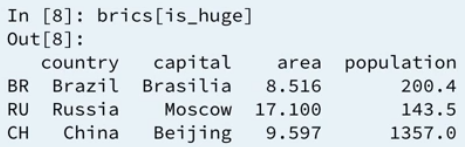It shows those countries with ares greater than 8 million km. The steps can be shorten into 1 line of code:

```brics[brics["area"] > 8]
```

Also, it is able to work with Boolean operators (np.logical_and(), np.logical_or() and np.logical_not()). For example, if it looks for areas between 8 and 10 km, then the single line code can be:

```brics[np.logical_and(brics["area"] &gt; 8, brics["area"] < 10)]
```

The result returns from the above code is Brazil and China.

## Intermediate Python for Data Science IThe subjects in this DataCamp’s track, Intermediate Python for Data Science include:

• Matplotlib
• Dictionaries and Pandas
• Logic, Control Flow and Filtering
• Loops

It looks at data visualization – how to visualize data, data structures – how to store data. Along the way, it shows how control structures customize the flow of your scripts (codes).

### Data Visualization

It is one of the key skills for data scientists and Matplotlib makes it easy to create meaningful and informative charts. Matplotlib allows us to build various charts and customize them to make it more visually interpretable. It is not an hard thing to be done and it is pretty interesting to work on it. In my previous write-up, I wrote about how to use Matplotlib to build a line chart, scatter plot and histogram.

Data visualization is a very important part in data analysis. It helps to explore the dataset which it extracts insights. I call this as data profiling, the process of examine the dataset coming from existing data source such as databases, which consists of statistics or summaries of the dataset. The purpose is to find existing data can be used for other purposes, determine the accuracy, completeness and validity of the dataset. I can relate this to “perform a body check on the dataset to ensure it is healthy”.

One of the methods I learned from my school on data profiling is the use of histogram, scatter plot and boxplot to examine the dataset and find out the outliers. I can use either the Python’s Matplotlib, Excel, Power Bi or Tableau to perform this action.

### It does not end here…

Python allows us to do customization on the charts to suit our data. There are many types of charts and customization ones can do with Python, changing from colours, labels and axes’ tick size. It depends on the data and the story ones want to tell. Refer the links above to read my write-up on those charts.

### Dictionaries

We can use lists to store a collection of data and access the values using the indexes. It can be troublesome and inefficient when it comes to large dataset, therefore, the use of dictionaries in data analysis is important as it represents data in the form of key-value pairs. Creating a dictionary from the lists of data can be found in this link. It has one simple example demonstrating how to convert it. However, I do have a question, how about converting long lists to dictionary? I assumed it is not going to be the same method in this simple example. Does anyone have an example to share?

If you have questions about dictionaries, then you can refer to my blog which I wrote a quite comprehensive introduction of dictionaries in Python.

### What is the difference between lists and dictionaries?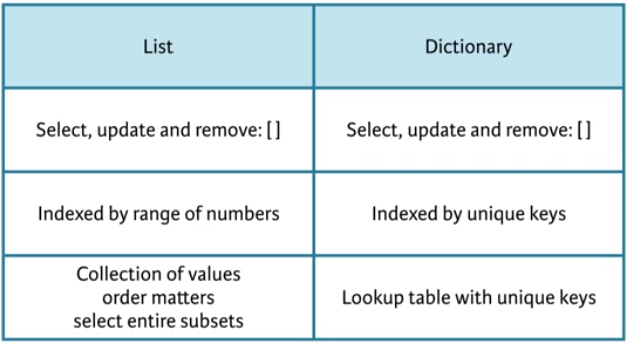If you have a collection of values where order matters, and you want to easily select entire subsets, you will want to go with a list. On the other hand, if you need some sort of lookup table where looking for data should be fast, by specifying unique keys, dictionary is a preferred option.

### Lastly, Pandas

Pandas is a high level data manipulation tool built on top of NumPy package. Since NumPy 2D array allows to use one data type in their elements, it may not suitable for some of the data structure which comprise of more than one data type. In Pandas, data is stored like a tabular table called DataFrame, for example: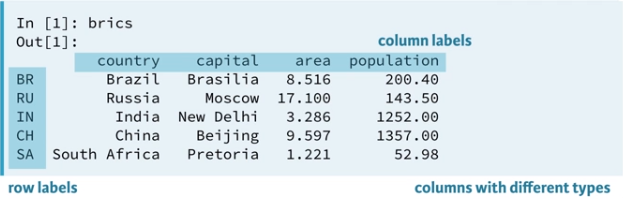### How to build a DataFrame?

There are few ways to build a Pandas DataFrame and we need to import Pandas package before we begin. In my blog, there are two methods shared, using dictionaries and external file such as .csv file. You can find the examples from the given link. Reading from dictionaries can be done by converting dictionary into DataFrame using DataFrame() and reading from the external file can be done using Pandas’ read_csv().

• Converting dictionary using DataFrame()
• reading from external file using read_csv()

### How to read from a DataFrame?

The above screenshot shows how the Pandas’ DataFrame looks like, it is in the form of rows and columns. If you wonder why the first column goes without naming. Yes, in the .csv file it has no column name. It appears to be an identifier for each row, just like an index of the table or row label. I have no idea whether the content of the file is done with this purpose or it has other meaning.

### Index and Select Data

There are two methods you can select data:

• Using square bracket []
• Advanced methods: loc and iloc.

The advanced methods, loc and iloc is Python’s powerful, advanced data access. To access a column using the square bracket, with reference to the above screenshot again, the following codes demonstrate how to select that country column:

```brics["country"]
```

The result shows the row label together with the country column. This is how it read a DataFrame which it returns an object called Pandas Series, which you can assume Series is a one dimension labelled array and when a bunch of Series comes together then, it is called DataFrame.

If you want to do the same selection of country column and keep the data as DataFrame, then using the double square brackets, it can do the magic with following code:

```brics[["country"]]
```

If you check the type of the object, it returns as DataFrame. You can define more than one column to be returned. To access rows using the square bracket and slices, with reference to the same screenshot, the below code is used:

```brics[1:4]
```

The result returns the from row number 2 to 4 or index 1 to 3 which contains, Russia, India and China. If you still remember, the characteristic of slice? The stop value (end value) of a slice is exclusive (not included in the output.

However, this method is has a limitation. For example, if you want to access the data similar to 2D Numpy Array, it can be done using the square bracket with specific column and row.

```my_array[column, row]
```

Hence, Pandas has this powerful and advanced data access, loc and iloc, where loc is a label based and iloc is position based. Let us looking into the usage of loc. The first example reads row loc and follow by another example reads row and column loc. With the same concept as above, single square bracket returns a Series and double square brackets return a DataFrame, just as below:

```brics.loc["RU"] --Series single row
brics.loc[["RU"]] --DataFrame single row
brics.loc[["RU, "IN", "CH"]] --DataFrame multiple row
```

Let extends the above code to read country and capital columns using the row and column with loc. First part it mentions the rows and second part it mentions the column labels. The below code returns a DataFrame.

```brics.loc[["RU, "IN", "CH"], ["country", "capital"]]
```

The above rows values can be replaced with slice, just like the sample code below:

```brics.loc[:, ["country", "capital"]]
```

The above code did not specify the start and end index, it means it returns all the rows with country and capital columns. Below is the screenshot of comparison between square brackets and loc (label-based).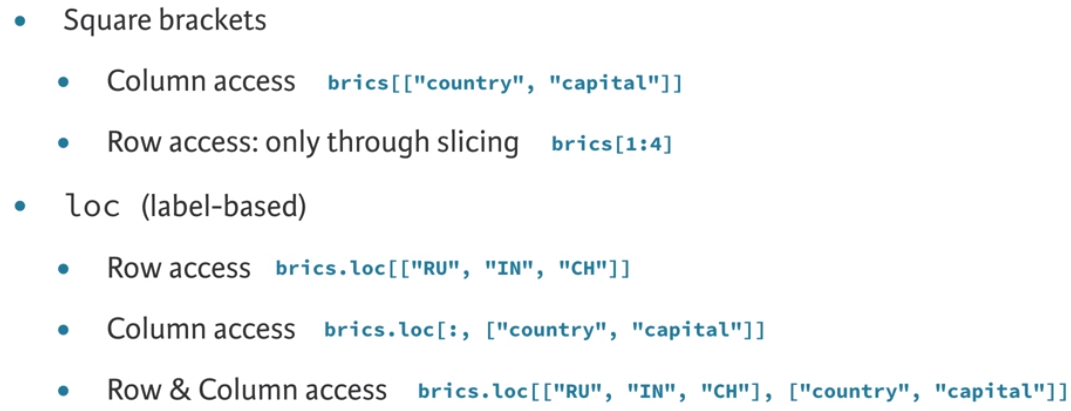Using iloc is similar to the loc, the only different is how you refer column and row which is using index instead of specifying the rows and column labels.

## Python: formatterBelow shows how to do more complicated string formatting. Refer below for the sample code:

```formatter = "{} {} {} {}"

print(formatter.format(1,2,3,4))
print(formatter.format("one","two","three","four"))
print(formatter.format(False, False, True, False))
print(formatter.format(formatter, formatter, formatter, formatter)
print(formatter.format(
"First thing",
"that we can try",
"maybe is having",
"a line of sentence"))
```

It is using something called function to turn the formatter variable into other strings. When the code write, formatter.format, it tells the Python compiler to do the following:

• Take its formatter string declare in the first line.
• Call its format function.
• Pass to format function, the 4 arguments which matches up with the 4 curly brackets {}s in the formatter variable.
• The result of calling format on formatter is the new string that has the {} replaced with four variables.

This is what the print statement prints.
1,2,3,4
one, two, three, four
False, False, True, False
{} {} {} {} {} {} {} {} {} {} {} {} {} {} {} {}
First thing that we can try maybe is having a line of sentence

## Python: Introduction IVIt has been a while back I wrote about Python Introduction I, II and III. Today, I am going to complete the last part of the introduction, the NumPy. Months ago during my Python’s self-learning time, I wrote about NumPy, here is the link.

### NumPy

It is an alternative to Python List, the NumPy array helps us to solve problems dealing with Python List’s operations. Calculations on Python Lists cannot be done in the same way we do for two integers or strings. This package needs to be installed before we can import and use it.

In my blog above, I wrote about the behaviour of the NumPy Array. It does not allow different types of elements in the array. When a NumPy Array is built, element’s data type changed to end up with a homogeneous list. Supposed, the list contains a string, number and Boolean, now it changes to all string format, for an example.

Also, the operator “+”, “-” and etc which we used along with Python List, are different in NumPy Array. Refer below for an example:

```py_list = [1,2,3]
numpy_array = np.array([1,2,3])
py_list + py_list
numpy_array + numpy_array
```

First output shows the two lists are merged or combined together into a single list. Second output shows an array returns an output of addition of those numbers. The screenshot below shows the result which I used Jupyter Notebook to execute.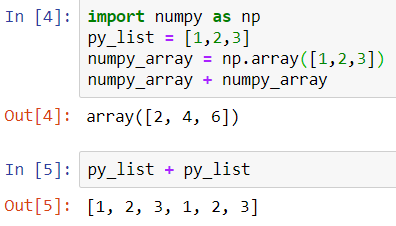Whatever that it has covered in the link above is good enough to give us a basic understanding of Numpy. If you wish to learn more, there is another link I found from the Medium which we can refer to.

### NumPy Subsetting

Specifically for NumPy, there is a way of doing list subsetting, using an array of Boolean. Example below shows how we can get all the BMI values above 23. Refer to the example from DataCamp,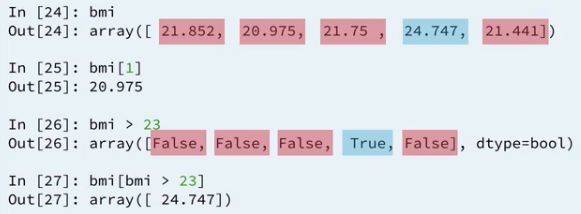First result returns as Boolean, True if BMI value is above 23. Then, you can use this Boolean array inside a square bracket to do the subsetting. When the Boolean’s value is True, it selects its value.

In short, it is using the result of the comparison to make a selection of data.

### 2D NumPy Array

I covered the 2D NumPy Array in this link, where it shows how to declare a 2D NumPy Array and how does it work in subsetting, indexing, slicing and perform math operations.

### NumPy: Basic Statistics

You can generate summary statistics of the data using NumPy. Python NumPy has few useful statistical functions which can be used for analytics. It includes finding min, max, average, standard deviation, variance and etc. from a given elements in the array. Refer to my write up on this basic statistics in this link.

## Python: Introduction IIIThe last part of the Python Introduction and I will cover topics on functions, methods and the packages in Python. For sure, there is a difference between function and method. I revisit my original post which I wrote about the differences between functions and methods. You can read up those before continue here.

## User-defined Functions

The simplest way I can explain what is function which I wrote in my original post:

A function is a block of code to carry out a task and it calls by its name. All functions may have zero or many arguments. The arguments are passed explicitly (directly). On the exit of the function, it may or may not return value or values.

There are some examples in this post to explain about functions, how to define a function with and without arguments, uses default value for an argument, uses flexible arguments *args and **kwargs and uses of return statement in the function.

## Methods

It is like a function, except it is attached to an object (dependent). A method is implicitly (indirectly) passed to the object for which it is invoked. It may or may not return a value or values. The method is accessible to data that is contained within the class.

For methods examples, I wrote it in this post.

## Packages

Think the packages as a directory of Python scripts. Example each .py script is a module. This module specifies functions, methods and types in solving a particular problem. I found a link which explained in detail about packages in Python. Refer here for more reading.

In this part III, I know there are many external links are given, mainly is to reduce re-write of those entries which I wrote them sometimes ago. This blog serves as a place to find the relevant resources for reading and examples which I think it is enough to cover the basic understanding of the functions, methods and packages in Python.

## Python: Introduction III continue from the Python: Introduction which I wrote it yesterday and it gave the very basic idea of how Python is in term of declaring variables, the data types and how we can store the data in a collection. So. variable, data type and collections. If you missed out or cannot recall them, here is the link to yesterday’s post. There are links to various data types and the collections. I did not want to repeat here.

For the part 2, I have decided to concentrate on writing the introduction to control flows. I think it will be great to have these control flows being taught first before we head to more Data Science orientated topic such as using the package called Numpy and creating our own functions and methods. So, I swapped a little bit in term of syllabus or topic in my writing.

## if, elif, else

It is a conditional statement which we can use to match certain conditions. There are few methods to write this statement and it is not always that we need to use elif and else. Let us look at this syntax below:

```if condition :
expression
```

This syntax has 1 single condition to match only, that is why “if” is being used. Example:

```z = 4
if z % 2 == 0 :
print("z is even")
```

All control flows has a standard syntax and indentation applied to each of them to mark the beginning to the expression or what should it does when the condition is matched. Therefore, you can see the print() statement is slightly indented. In most IDEs, it is automatically indented when we use the colon (:) sign after the condition, in this case, z % 2 == 0.

The moment we have 1 more condition in our code, if else statement is used. See the syntax below:

```if condition :
expression
else :
expression
```

In the else statement, often we do not need to specify the condition within the line because it is understood that when the if statement or condition did not match then it goes to the else statement and execute the code. It looks as though else statement is a default statement. I know in some other programming language, it does have default statement at the end of else statement which say, anything not matches then run default statement.

We can omit this else statement when we do not require to process anything if the first condition does not match. However, sometimes, we might miss out important scenarios of the if statement is skipped. What I meant here is besides being legit that the condition does not match, there is also possibilities of exceptions happened while checking through the conditions. It is recommended to use the else statement as an exception handling, either print out a line in the console or log file. This helps in the debugging procedure.

```z = 5
if z % 2 == 0 :
print("z is even")
else
print("z is odd")
```

The above is an example of using if-else statement when there are two conditions, either-or situation. The variable z is 5, hence it goes to the else statement and print out “z is odd”.

Next, if-elif-else statement is used when there are few conditions in the scenario which one may get matched during the condition checking. When the first condition does not match, it goes to the next elif condition to check until it has no matches, it will end at else statement. You can have many elif statements in your codes. Below is the syntax:

```if condition :
expression
elif condition :
expression
else :
expression
```

Example of using the syntax:

```z = 3
if z % 2 == 0 :
print("z is divisible by 2")
elif z % 3 == 0 :
print("z is divisible by 3")
else :
print("z is neither divisible by 2 nor by 3")
```

The output is z is divisible by 3. After each expression, the statement terminates and returns the result. It will not proceed to check whether next condition is matched. With these 3 examples, I hope it gives some ideas about if statement, if-else statement and if-elif-else statement.

## while

while statement works by repeating an action until condition is met. It is important to assess the code before running the while statement because if any chances the condition is not met, the statement will keep running and this we call it infinite loop. You have to force to end the application manually. The syntax for while statement:

```while condition :
expression
```

Example of using the while syntax:

```x = 0
while x < 5:
print(f'The number is {x}')
x += 1
```

The most crucial part here is the variable x which works as a counter to ensure the condition is met. Without this line of code (x += 1 ) the condition is always true and the loop becomes infinite.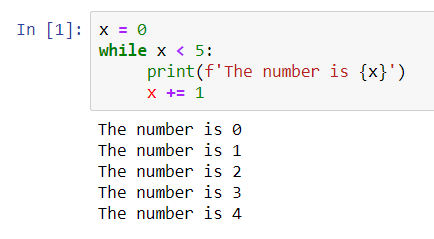This is the output from executing the while statement. When x = 5, it stops and exits from the while statement and does not print out anything.

## for

Remember in the previous blog, I mentioned about using Python list (collections)? For statement is a good control flow to iterate (repeat) through the Python list to get each element.

```for var in seq :
expression
```

Without using for statement, we might want to repeat few times of the print statements to print out the elements inside the Python list below:

```fam = [1.73, 1.68, 1.71, 1.89]

print(fam)
print(fam)
print(fam)
print(fam)
```

Although this is a correct syntax, it is not a good practice. Below demonstrates how to use a for statement to iterate through the Python list and print the values out.

```for height in fam :
print(height)
```

Both of the codes returns the same output.

```1.73
1.68
1.71
1.89
```

For statement works well for any types of collections or even with a string. Below example uses my_string as the “list” and a variable character as the “item_name” to represent the elements inside the list. One by one, it prints out each character in the my_string.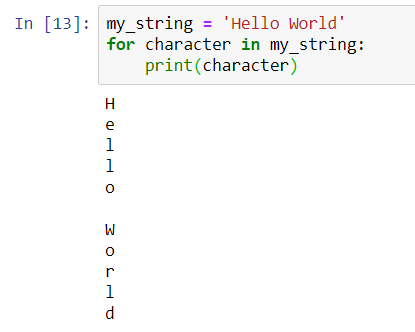In for statement, we can use the enumerate(), a Python built-in function. enumerate() method adds a counter to an iterable and returns it in a form of enumerate object. This enumerate object can then be used directly in for loops or be converted into a list of tuples using list() method.

### Why is enumerate return a tuple?

When enumerate() returns in a form of enumerate object, it comes in a form of index, value. It is because enumerate() accepts start parameter which is the index value of the counter, by default it is 0. A simple illustration is as below:

```enumerate(iterable, start=0)
```
```list = ["eat","sleep","repeat"]
print(enumerate(list)
```

When we check the output from the console, it shows as below:

```[(0, 'eat'), (1, 'sleep'), (2, 'repeat')]
```

It starts with an index 0, of course, it can be changed with indicating the index value, such as enumerate(list,1), then the index begins with 1 instead of 0. This enumerate() function may look useful when we want to list the elements from the collections with the index and value.

```fam = [1.73, 1.68, 1.71, 1.89]
for index, height in enumerate(fam) :
print("index " + str(index) + ": " + str(height))
```

Reusing the above example and now, we have added enumerate(fam) in the for statement instead of using “for height in fam”. Then, in the print() statement, we convert the index value and height value to string and concatenate them. This maybe useful when we want to print out our shopping cart’s items list. Its output shows:

```index 0: 1.73
index 1: 1.68
index 2: 1.71
index 3: 1.89
```

Mastering the use of the control flows can help in the later stage when we go into the data structure section. I have written separate blogs about if-else statements, while and for loops, you can refer to the links below:

## Python: Introduction IIt has been a while I stopped learning Python from DataCamp due to my part time classes and assignment, and work commitment. It is not easy to keep track each of them everyday. On top of it, I still have my volunteer work with TechLadies and regularly have to meet up to brainstorm and updates each other.

Today’s topic is very much on Python, definitely. I want to concentrate on my writing in Python for next 2 weeks before I head off for an holiday. I am sure, I will be lazy after my break. It would be great if I can write up something to summarize or reorganize what I have been writing for the past few months on my Python’s learning using DataCamp and Udemy.

Remember my very first day I started learning Python using Udemy, it taught about the installation and I went on to install IntelliJ. Till to date, I hardly using it, most of my time, I am using the online version of Jupyter Notebook. I find it pretty easy to be used. I understood that there are many other IDEs in the market and there is no specific software to be used to code Python. For now, I will just keep it simple for my learning.

## How to begin?

After the installation of the python 3, I open the terminal (in Linux) or command prompt (Windows) to go into the Python’s shell by typing the following command:

```python
```

From the terminal or command prompt screen, I can see a return message from Python with the version number. There are Python 2 and Python 3. So, be clear on which version is being used on the machine because the syntax are slightly different from each others.

## Checking version

On the very first time, we always want to know if everything we installed for Python works or not. Checking the version, if it is updated, latest and correct version to be used is first time we might want to do with:

```python --version
```

Simple open up your terminal or command line to type the above command on it. On the screen, it may return you the version info such as below:

```Python 3.7.0
```

## print(‘Hello World’)

Next, we always start with simple print statement using the built-in function named print() to print out some lines, most often we will print in our first line is “Hello World”. Really, most people who first started learning programming language will have this line printed. I use this function everywhere in my coding and it is very useful. It is just same as the PRINT statement from the SQL server, if you are coming from database background. Using single quote or double quote is not a matter.

```print('Hello World!')
print("Hello World!")
```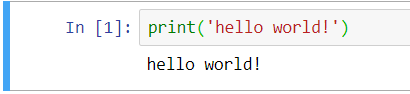## Variables and Types

Then, we touch on the variables and types, the important component in most programming languages. Variables and types are interrelated. I discussed about the characteristic of a variable in my first post. Let me have it here too!

• Specific and case-sensitive name, best practice to use lowercase.
• Define things that are subject to change.
• Can be used to store texts, numbers or dates.
• Cannot use space  and symbols in the name, use _ instead.

Then, there are plenty of different data types as well, yes, that is the types I meant here. Remember, different types have different behaviours. I wrote many posts about each of them before. I will link them up whenever we re-visit the topic.

• Boolean operations: and, or, not (True, False)
• Numeric types: int, float, complex (number, decimal)
• Text sequence type: str (string)
• Sequence type: list, tuple, range
• Mapping type: dict
• Sets type: set
• None is frequently used to represent the absence of a value, as when default arguments are not passed to a function. It is a null value or no value at all which is different than empty string, 0 or False.

The simplest way to demonstrate how we can create a variable and assign a value to it.

```height = 1.67
weight = 180

name = 'Joanne'
gender = 'Female'

isStudent = True
```

The above shows the height and weight variables in float and int data type, then we have name and gender in string and a variable called isStudent with a Boolean value. In Python, it does not require to declare a variable with any prefix in front of or behind the variable which we can see in Javascript or SQL Server, if you are familiar with those languages. Then, you may ask how does compiler (computer) knows it is of what type of data types.

## What is the difference between (=) and (==)?

The single equal sign (=) assigns the value on the right to a variable on the left whereas the double equal sign (==) tests if the two things have the same value. The two things can be a comparison of two variables or a variable with a math operator.

## type()

```type(height)
type(weight)
type(name)
type(isStudent)
```

type() is a built-in function which allows us to check the data types of the variables we created with assigned values. type() helps to answer the above question.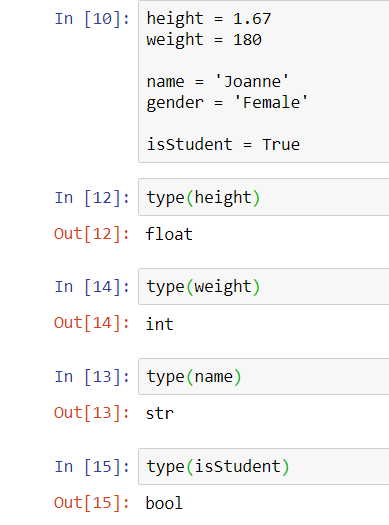That completed the fundamental and basic to code in Python. Now, you know how to do the following:

• use the print() statement to print texts.
• use of variables and data types.
• use the type() statement to print out the data type of a variable.

Probably, now you want to know what is integer, string, Boolean and etc. I have some links here to help out the basic explanation together with examples:

To talk about numbers and strings, it can be another topics by its own as there are many interesting about them such as the use of (+) sign. It is concatenate sign which means it combines two or more variables of same type together. The way number and string use (+) sign also difference than each other. Also, we have to remember that in Python, string and integer cannot use of (+) sign together. It throws exception (error). Exception is a programming jargon means error. There is a topic of exception handling in Python too. In this case, there is string formatting and integer formatting.

Let us move into fundamental part two, Python List.

## Python Collections

It is an interesting topic and important part in Python. Almost everyone of us will use Python List in our daily coding life 🙂 It is a collection of values and allows to have different types within the elements, one of the most simplest and easiest collections. When it comes to the word “collection”, Python has four type of collections.

You can read more about the basic of these collection here. Each of them has different characteristics, syntax, structure and usage. Along the way, we use different collections to explain the Python codes and concepts. Below is an example of how list looks like:

```fruits = ['orange', 'apple', 'pear', 'banana', 'kiwi', 'apple', 'banana']
```

Declaring a list is same as declare a variable, it just requires to follow the list’s syntax to create one. As mentioned earlier, it can be any data types in a list. So, you can declare a list as below too:

```family = ['Anna', 1.73, 'Eddie', 1.68, 'Mother', 1.71, 'Father', 1.89]
```

We can use the lists above to work with control flows, going through the iteration and/or condition checking, then calculate a value and return a result. I think I will cover it in the next post.

Up to now, this portion is still a basic Python and does not involve any analytics or data science work if you are looking for one.